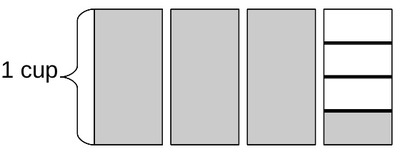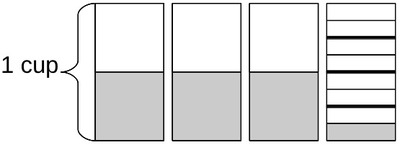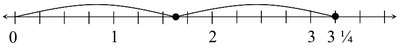# Half of a Recipe

Alignments to Content Standards: 5.NF.B.6

Kendra is making $\frac{1}{2}$ of a recipe. The full recipe calls for $3\frac{1}{4}$ cup of flour. How many cups of flour should Kendra use?

## IM Commentary

This is the third problem in a series of three tasks involving fraction multiplication that can be solved with pictures or number lines. The first, 5.NF Running to school, does not require that the unit fractions that comprise $\frac{3}{4}$ be subdivided in order to find $\frac{1}{3}$ of $\frac{3}{4}$. The second task, 5.NF Drinking Juice, does require students to subdivide the unit fractions that comprise $\frac{1}{2}$ in order to find $\frac{3}{4}$ of $\frac{1}{2}$. This task also requires subdivision and involves multiplying a fraction and a mixed number.

Note that the context here involves volume. While the picture can be drawn to look something like measuring cups, the number line representation is more abstract relative to the context. This helps transition students to a more abstract understanding of fraction multiplication.

## Solutions

Solution: Drawing a Picture

The first picture represents $3\frac{1}{4}$ cup of flour.The second picture represents $\frac{1}{2}$ of $3\frac{1}{4}$ cup of flour.Kendra should use $\frac{1}{2}$ + $\frac{1}{2}$ + $\frac{1}{2}$ + $\frac{1}{8}$ = $\frac{13}{8}$ = $1\frac{5}{8}$ cup of flour.

Solution: Using a Number Line

First plot a point at $3\frac{1}{4}$ to represent the amount of flour in the whole recipe.If we mark half way between 0 and $3\frac{1}{4}$, we see the point is half-way between $1\frac{1}{2}$ and $1\frac{3}{4}$.We can identify what point this is by putting tick marks between each of the tick marks representing fourths.

This way we can see that $1\frac{5}{8}$ is half-way between 0 and $1\frac{3}{4}$. So Kendra should $1\frac{5}{8}$ cup of flour.

Solution: Computing a Product

Since we want to know what $\frac{1}{2}$ of $3\frac{1}{4}$ is, we can multiply: \begin{equation} \frac{1}{2} \times 3\frac{1}{4} = \frac{1}{2} \times \frac{13}{4} = \frac{1 \times 13}{2 \times 4} = \frac{13}{8} = 1\frac{5}{8} \end{equation} Kendra should put $1\frac{5}{8}$ cups of flour.

Solution: Use the Distributive Property

A student could solve by multiplying $\frac{1}{2}$ by $3 \frac{1}{4}$, using the distributive property to avoid rewriting $3 \frac{1}{4}$ as an improper fraction.

\begin{align*} \frac{1}{2} \left( 3 + \frac{1}{4} \right) & = \frac{1}{2} \times 3 + \frac{1}{2} \times \frac{1}{4}\ & = \frac{3}{2} + \frac {1}{8} \ & = 1 \frac{1}{2} + \frac {1}{8} \ & = 1 \frac{4}{8} + \frac{1}{8}\ & = 1 \frac{5}{8} \end{align*}

This strategy should be compared side-by-side with the "Draw a Picture" solution above. Taken together, these solutions represent essentially the same strategy represented pictorially and numerically.

Solution: Use Decimal Numbers

If the student recognizes that this problem is calling for a product of fractions, he or she could approach this problem by converting to decimals and finding the product of $0.5$ and $3.25$. Now

$$3.25 \times 0.5 = 1.625$$

So that Kendra needs $1.625 = 1 \frac{5}{8}$ cups of flour.